Module 24 - Vectors

Introduction | Lesson 1 | Lesson 2 | Lesson 3 | Self-Test

Lesson 24.3: Projectile Motion

It is useful to find a vector function that models the path of a projectile. Such a function can yield the particle's position at time t as well as it's velocity and acceleration. This lesson will explore finding the function that models the path of such an object given some initial information.

Finding the Position Vector

A projectile is fired from ground level. If the starting point for the projectile is considered to be the origin and if air resistance is neglected, the position vector for the projectile at time t is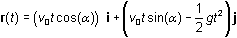where v0 is the initial velocity of the projectile,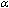is the angle from the horizontal at which the projectile is fired and g is the acceleration due to gravity.

The position vector can also be represented with the parametric equations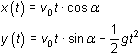Exploring a Specific Example

Suppose a projectile is fired at an angle of= 20° from the horizontal with an initial velocity of 180 ft/sec. Graph the path of the projectile using 32 ft/sec2 for g.

• Switch to Degree mode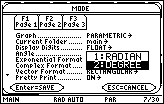• Set xt1(t) = 180*t*cos(20)
• Set yt1(t) = 180*t*sin(20) – (1/2)*32*t^2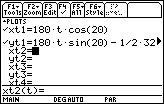• Enter the following window values• Graph the path of the object

24.3.1 Use the trace key to approximate when the projectile reaches its highest point and the maximum height. Click here for the answer.

Recalling Previous Functions

If you have not cleared the variables r,v and a since Lesson 24.2, these variables should still be defined as the position, velocity and acceleration vectors for the parametric equations stored in xt1 and yt1. If you have cleared these variables, redefine them as they were defined in Lesson 24.2.

• Define r(t) = [xt1(t), yt1(t)]
• Define v(t) = d (r(t), t)
• Define a(t) = d (r(t), t, 2)

Verify that these functions have been updated with the new parametric equations

• Enter r(t)
• Enter v(t)
• Enter a(t)Notice the acceleration vector is 0i - 32j for all values of t because the only force acting on the projectile is gravity.

Finding the Highest Point

Find the vertical component of the velocity vector.

• Enter v(t)[1,2]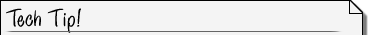The TI-89 treats vectors as though they are 2-dimensional arrays that just happen to have only one row. The notation v(t)[1, 2] represents the component in the first row, second column of the velocity vector, which is the vertical, or height, component.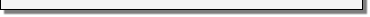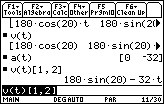When the projectile reaches its highest point the vertical component of the velocity vector is zero.

24.3.2 Find when the projectile reaches it highest point by entering

solve(v(t)[1,2] = 0, t)